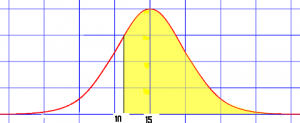# Q Function: Definition, Examples

Share on

In statistics, the Q function Q(x) usually refers to the normal distribution function Φ(x). For other meanings, see Other Uses.

## The Normal Distribution Q Function Φ(x)

The Q function is just one minus the cumulative distribution function (CDF) for the standardized normal distribution. In other words, it gives you the right tail area.The area of a right tail in the normal distribution (yellow area) can be found with the Q function.

The CDF for the normal distribution gives you the probability that a normal random variable takes a value equal to or smaller than x. The Q function is the complement of this; In other words, it’s the probability a normal random variable takes a value greater than x.

As a formula:
Q(x) = 1 – CDF = P(X > x)

The plot starts with an area of 1, representing 100% probability. At the point on the far left of the bell curve, the right “tail” is actually the entire area of the curve.

Calculating the function by hand is relatively simple: find the CDF, and subtract from one. Some software programs find the Q function directly. For example, In MATLAB, the syntax is y = qfunc(x). If your software doesn’t, find the CDF and subtract from one.

## Other Meanings

There are other meanings for the Q function, including:

## References

Gupta, M. & Chen, Y. (2011). Theory and Use of the EM Algorithm. Foundations and Trends in Signal Processing. Vol. 4, No. 3 (2010) 223–296. Retrieved December 6, 2017 from http://www.mayagupta.org/publications/EMbookGuptaChen2010.pdf

CITE THIS AS:
Stephanie Glen. "Q Function: Definition, Examples" From StatisticsHowTo.com: Elementary Statistics for the rest of us! https://www.statisticshowto.com/q-function/
---------------------------------------------------------------------------Need help with a homework or test question? With Chegg Study, you can get step-by-step solutions to your questions from an expert in the field. Your first 30 minutes with a Chegg tutor is free!